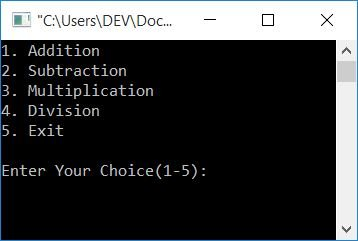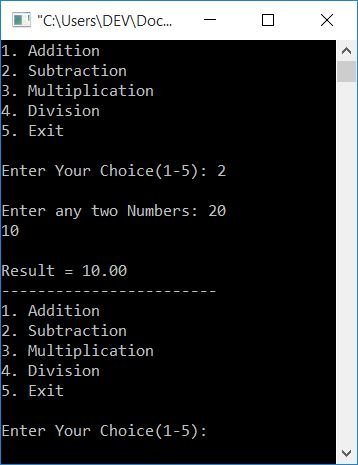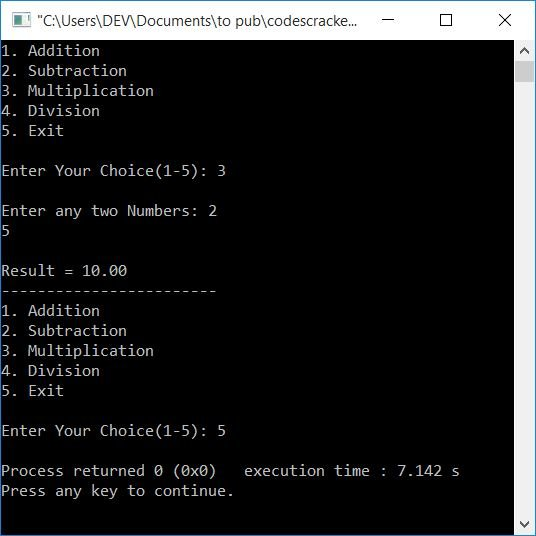# C Program to Make a Simple Calculator

In this article, you will learn about creating a simple calculator program in C. A simple calculator is a calculator that performs four basic mathematical operations such as addition, subtraction, multiplication, and division. Here, the calculator program is created in two ways:

In C programming, to make a simple calculator that can do the four most basic math operations, use the switch case to figure out which input operator to use for each calculation, as shown in the program below.

## C Calculator Program with Switch Case

Here is a simple menu-driven program based on simple calculations like addition, subtraction, multiplication, and division according to the user's choice:

```#include<stdio.h>
#include<conio.h>
int main()
{
float num1, num2, res;
int choice;
do
{
printf("2. Subtraction\n");
printf("3. Multiplication\n");
printf("4. Division\n");
printf("5. Exit\n\n");
scanf("%d", &choice);
if(choice>=1 && choice<=4)
{
printf("\nEnter any two Numbers: ");
scanf("%f %f", &num1, &num2);
}
switch(choice)
{
case 1:
res = num1+num2;
printf("\nResult = %0.2f", res);
break;
case 2:
res = num1-num2;
printf("\nResult = %0.2f", res);
break;
case 3:
res = num1*num2;
printf("\nResult = %0.2f", res);
break;
case 4:
res = num1/num2;
printf("\nResult = %0.2f", res);
break;
case 5:
return 0;
default:
printf("\nWrong Choice!");
break;
}
printf("\n------------------------\n");
}while(choice!=5);
getch();
return 0;
}```

This program was built and runs in the Code::Blocks IDE. Here is the initial snapshot of the sample run:Now supply any input (as a choice from 1 to 5) in a way that:

• Enter 2 for subtraction.
• Enter 3 for multiplication.
• Enter 4 for Division.
• and enter 5 to exit.

Let's suppose the user enters 2 as his/her choice, so the program will ask again to enter any two numbers to perform the subtraction. For instance, if the user enters 2 as a choice and 20 as the first number, followed by 10 as the second number. Press the ENTER key to see the output as shown in the snapshot given below:Press 5 to exit the calculation process. Let's take a look at the explanation of this program.

#### Program Explained

• Because a do-while loop executes its statement first, it checks the condition (at the end of the loop's body) after running all the statements placed inside the block of this loop.
• So, program flow enters into the do body, and all five menus get printed automatically.
• And then the program asks the user to enter his or her choice from 1 to 5.
• Let's suppose if the user enters 2 as input, 2 gets initialized to the choice variable.
• Because the value of choice is greater than or equal to 1 and less than or equal to 4,
• So program flow goes inside the if block and scans two numbers from the user.
• Let's suppose the user enters two numbers, say 20 and 10.
• Now, using the switch case, program flow goes inside its block and creates all 5 cases with their corresponding operations, that is, from addition to exit.
• Because the user enters 2 as input, case 2's statement gets executed.
• As a result, num1-num2 or 20-10 or 10 is assigned to the res variable.
• And the value of res gets printed as output, which is the subtraction result of the two given numbers.
• Then use the break statement to go to the condition part of the do-while loop and check whether the value of choice is not equal to 5 or not.
• If it is not equal to 5, then program flow again goes back to the start of the "do-while" loop's body.
• Otherwise, if it is equal to 5, then program flow exits from the do-while loop.
• Do the operation until the user enters 5 as his or her choice.

## Create a simple calculator using a C function

After understanding the above program, it is easy to create the same program using functions. So here is the calculator program that uses functions in C.

```#include<stdio.h>
#include<conio.h>
float subFun(float, float);
float mulFun(float, float);
float divFun(float, float);
int main()
{
float num1, num2, res;
int choice;
do
{
printf("2. Subtraction\n");
printf("3. Multiplication\n");
printf("4. Division\n");
printf("5. Exit\n\n");
scanf("%d", &choice);
if(choice>=1 && choice<=4)
{
printf("\nEnter any two Numbers: ");
scanf("%f %f", &num1, &num2);
}
switch(choice)
{
case 1:
printf("\nResult = %0.2f", res);
break;
case 2:
res = subFun(num1, num2);
printf("\nResult = %0.2f", res);
break;
case 3:
res = mulFun(num1, num2);
printf("\nResult = %0.2f", res);
break;
case 4:
res = divFun(num1, num2);
printf("\nResult = %0.2f", res);
break;
case 5:
return 0;
default:
printf("\nWrong Choice!");
break;
}
printf("\n------------------------\n");
}while(choice!=5);
getch();
return 0;
}
{
return (a+b);
}
float subFun(float a, float b)
{
return (a-b);
}
float mulFun(float a, float b)
{
return (a*b);
}
float divFun(float a, float b)
{
return (a/b);
}```

Here is a sample run of the above program:All four functions in this example accept two floating-point values as arguments, perform the operation, and then return the result to the main() function. That is, its return value gets initialized to the res variable.

#### The same program in different languages

C Quiz

« Previous Program Next Program »